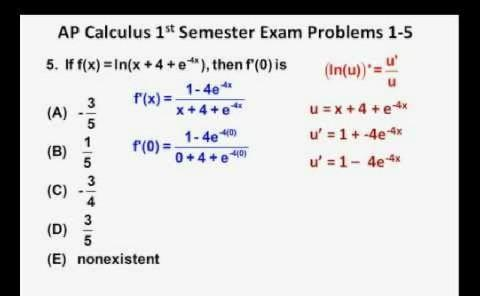# Are You Ready For An AP Calculus Practice Exam?

10 Questions | Total Attempts: 204SettingsAdvanced Placement Calculus is a set of two distinct Advanced Placement calculus courses and exams offered by College Board. AP Calculus AB covers limits, derivatives, and integrals. AP Calculus BC covers all AP Calculus AB topics plus additional topics (including more integration techniques such as integration by parts, Taylor series, parametric equations, polar coordinate functions, and curve interpolations). The test is not the actual AP. This prepares you for the actual test.

• 1.
If 4y+8 = 12y + 24, then y = ?
• A.

2

• B.

1

• C.

-1

• D.

-2

• 2.
If f(2) = 10 and f(4) = 44, which of the following could be f(x)?
• A.

2x+6

• B.

2x2+12

• C.

3x2- x

• D.

X-6x

• 3.
Line B contains points (3, 2) and (4, 5). If line V is perpendicular to line B, then which of the following could be the equation of line V?
• A.

Y = (-1/5)x + 3

• B.

Y = (- 1/3)x + 5

• C.

Y = - 3x + 5

• D.

Y = 5x + 1/3

• 4.
If the remainder when x is divided by 5 equals the remainder when x is divided by 4, then x could be any of the following except?
• A.

20

• B.

22

• C.

24

• D.

21

• 5.
If one packager can pack 15 boxes  every two minutes, and another can pack 15 boxes every three minutes, how many minutes will it take these two packagers, working together, to pack 300 boxes?
• A.

24

• B.

12

• C.

15

• D.

20

• 6.
The product of integers x and y is divisible by 36. If x is divisible by 6, which of the following must be true? i. y is divisible by x ii. y is divisible by 6 iii. y/6 is divisible by 6
• A.

None

• B.

I only

• C.

I and ii only

• D.

Ii only

• 7.
If x > 0 and [3 - √x] [3 + √x] = 7, what is the value of x?
• A.

4

• B.

3

• C.

2

• D.

1

• 8.
If -1 ≤ a ≤ 2 and -3 ≤ b ≤ 2, what is the greatest possible value of (a + b) (b - a)
• A.

7

• B.

12

• C.

6

• D.

9

• 9.
If the distance from (2, 6) to (1, b) is a,  a = [ | -1 | + 16 ]^1/2, and b < a, what is the value of b?
• A.

3

• B.

4

• C.

2

• D.

7

• 10.
If 3c + 2b + a = 22, b + a = 8 and a = 6, what is the value of c + b + a?
• A.

4

• B.

8

• C.

12

• D.

18

Related TopicsBack to top